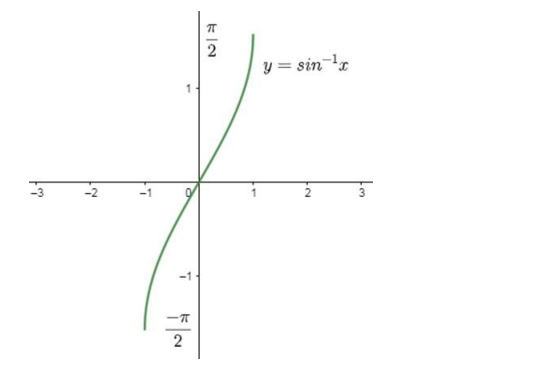# Write down the interval for the principal-value branch of each of the following functions and draw its graph:Question:

Write down the interval for the principal-value branch of each of the following functions and draw its graph:

$\sin ^{-1} x$

Solution:

Principal value branch of $\sin ^{-1} \mathrm{x}$ is $\left[-\frac{\pi}{2}, \frac{\pi}{2}\right]$